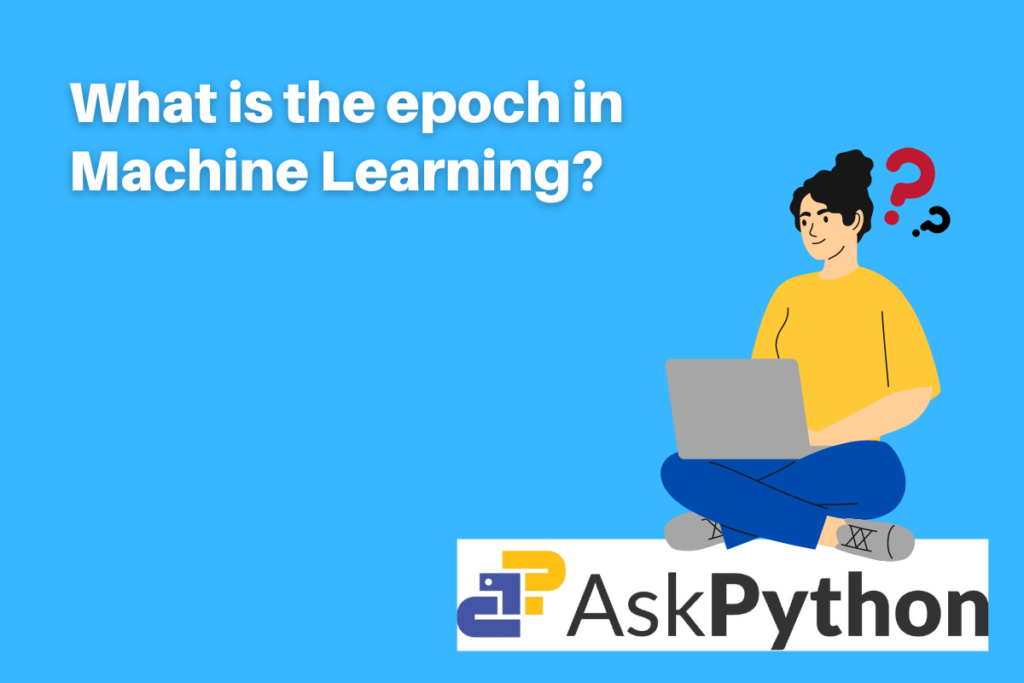# What is Epoch in Machine Learning?With the use of machine learning (ML), which is a category of artificial intelligence (AI), software programs can predict outcomes more accurately without having to be specifically trained to do so. Machine Learning has its own lingo and one should the key terminologies before starting with any machine learning or artificial intelligence project. One such very important term is “epoch”. In this article, let us try to understand what exactly is an epoch and why is it used in machine learning.

## Introduction to Epoch

Before we start with definitions, firstly let us understand the basic working concept of machine learning algorithms. In simple words, the algorithm tries to ‘learn’ from the provided input data. With little to no human involvement, it operates by examining data and discovering patterns. Just like human being learns from their mistakes, the machine learning model also learns from its own errors that come out as a result. In order to increase the accuracy rate of the machine-learning model. The algorithm’s repetitive nature necessitates repeated results to achieve the best outcome.

Epochs, batches, batch sizes, and iterations are only necessary when the data is too large, which occurs frequently in machine learning and prevents us from sending all the data to the system at once. In order to solve this issue, we must break up the data into smaller chunks and input each component one at a time into our system, updating the weights of the neural networks at the conclusion of each phase to fit them into the input data.

## What is epoch?

In machine learning, an epoch refers to one complete iteration of the algorithm over the training dataset. The amount of complete runs of the whole training dataset during the algorithm’s training or learning process is specified in terms of epochs. The internal model parameters of the dataset are modified at each epoch. The number of this epoch is a crucial hyperparameter for the method.

Typically, there are several epochs—hundreds or thousands—which allow the learning method to continue until the model’s error has been suitably reduced. But the precise number of epochs to be passed depends on the quality of the dataset. Overfitting is a problem when a model generalizes poorly but fits the training instances perfectly. On the other hand, if the model didn’t learn the data sufficiently, it is said to be underfitting. One can develop line plots that display the error or skill of the model on the y-axis and the epochs along the x-axis as time, to understand problems like overfitting or underfitting. The term “learning curves” is occasionally used to describe these graphs.

Now that you have a clear idea of what epoch is. Let’s understand a few more terminologies before more forward with an example of the epoch. also, understand these terms have their own meanings and have differences in their meanings.

• Sample: Several rows of data make up a training dataset. A sample is one row of data. Instance, observation, and input vector are other synonyms for samples.
• Batch: The complete dataset cannot be loaded at once into the machine learning model. As a result, you split the dataset into N sections. Batch refers to this one section of the large dataset.
• Batch Size: The number of samples in one batch is known as batch size.
• Iteration: The number of batches required to finish one epoch is called an iteration.

## Example of Epoch

Let us consider the dataset has 1000 rows, which means 1000 samples. Now it’s hard to pass the entire dataset at once to the model. Hence, we break it into batches with batch sizes equal to 20. This concludes that one epoch has 50 batches. Each batch of twenty samples will result in an update of the model weights.

Suppose we run 100 epochs, then the entire dataset will be presented to or run through the model 100 times. 100 x 50 = 5000 batches altogether will be created during the training procedure.

## Summary

In conclusion, this article is great to help anyone studying deep learning or machine learning. We have comprehended a few important terminologies like epoch, batch, and iteration commonly used under the domain of AI or machine learning, To get more beginners’ information about machine learning, click here.

To learn from more such detailed and easy-to-understand articles on various topics related to machine learning and Python programming language in general, do click here!